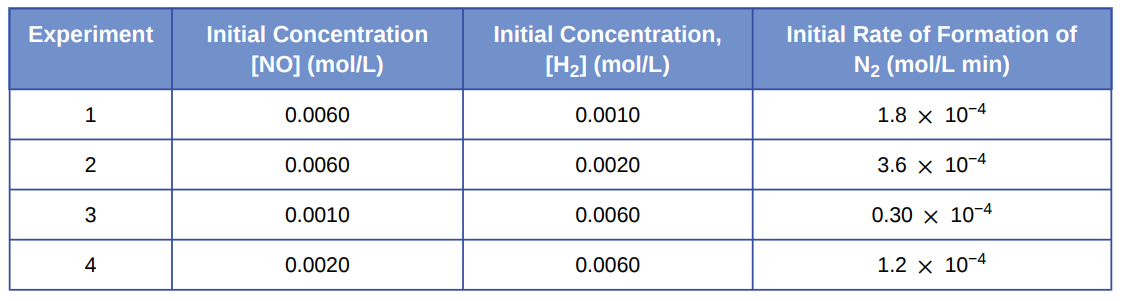# Problem: Experiments were conducted to study the rate of the reaction represented by this equation. 2 NO(g) + 2 H2(g) ⟶ N2(g) + 2 H2O(g) Initial concentrations and rates of reaction are given here.Consider the following questions:(d) For experiment 2, calculate the concentration of NO remaining when exactly one-half of the original amount of H2 had been consumed.

###### FREE Expert Solution

We’re being asked to calculate the concentration of NO remaining when exactly one-half of the original amount of H2 had been consumed.

Recall that the rate law only focuses on the reactant concentrations and has a general form of:

k = rate constant
A & B = reactants
x & y = reactant orders

We are going to do the following steps to solve this problem:

Step 1: Calculate the order of the reaction with respect to NO

Step 2: Calculate the order of the reaction with respect to H2

Step 3: Calculate the value of the rate constant

Step 4: Calculate the concentration of NO remaining

91% (401 ratings)###### Problem Details

Experiments were conducted to study the rate of the reaction represented by this equation.
2 NO(g) + 2 H2(g) ⟶ N2(g) + 2 H2O(g)
Initial concentrations and rates of reaction are given here.Consider the following questions:

(d) For experiment 2, calculate the concentration of NO remaining when exactly one-half of the original amount of H2 had been consumed.

Frequently Asked Questions

What scientific concept do you need to know in order to solve this problem?

Our tutors have indicated that to solve this problem you will need to apply the Rate Law concept. You can view video lessons to learn Rate Law. Or if you need more Rate Law practice, you can also practice Rate Law practice problems.

What textbook is this problem found in?

Our data indicates that this problem or a close variation was asked in Chemistry - OpenStax 2015th Edition. You can also practice Chemistry - OpenStax 2015th Edition practice problems.